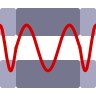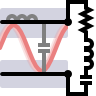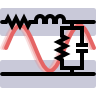# Transmission Lines

Transmission lines are very important historically since they enabled the communication over long distances. Other than that, they are very interesting from a didactical perspective:

• one-dimensional systems that can be characterized by very few parameters (in the end, it's only the impedance $$Z$$)
• very good example for slowly varying fields approximation, where $$\nabla\times\mathbf{B}\left(\mathbf{r},t\right)\approx\mu_{0}\mathbf{j}\left(\mathbf{r},t\right)$$ and thus the coupling of electric and magnetic field is solely given by Faraday's law $$\nabla\times\mathbf{E}\left(\mathbf{r},t\right)=-\dot{\mathbf{B}}\left(\mathbf{r},t\right)$$
• fundamental physical effects like wave propagation and reflection can be explained which will be a good bridge to complicated relativistic phenomena

With these facts in mind, we hereby dedicate a whole section to transmission lines.

## Fabry-Perot Resonances in a Transmission LineOne of the most important concepts in optics are Fabry-Perot resonances of certain cavities. Nevertheless, we can understand this resonance phenomenon on the basis of wave propagation in transmission lines! You can also find out how this principle is used in nanophotonics.

## Impedance Matching of Transmission Lines and Oscillator CircuitsFor technical applications it is extremely important to be able to transfer as much energy from a transmission line to some load. In this problem we will learn how this is achieved and understand the basic principles signal reflection and impedance matching.

## The Transmission Line - Deriving the Telegrapher EquationWe consider a transmission line in a lumped model. This will allow you to find the so-called telegrapher equation which describes the signal propagation along transmission lines in general. You will also see how such devices can even also be used as quantum bits.